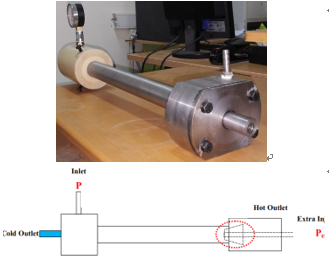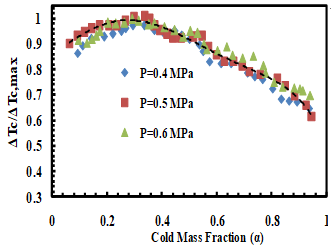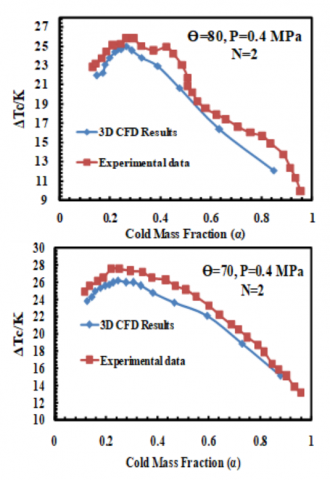# Experimental study on thermal performance of double circuit vortex tube (DCVT) - Effect of heat transfer controller angle

Experimental study on thermal performance of double circuit vortex tube (DCVT) - Effect of heat transfer controller angle

Department of Mechanical Engineering, Urmia University of Technology, Urmia P.O. Box 57122-419, Iran

Corresponding Author Email:
Page:
668-672
|
DOI:
https://doi.org/10.18280/ijht.350327
|
Accepted:
|
Published:
30 September 2017
| Citation

OPEN ACCESS

Abstract:

The patterns of heat and mass transfer can be changed using various heat transfer controller angle in Double Circuit vortex tube (DCVT). In this parametric/experimental test, the effective parameter is focused on the heat transfer controller angle in DCVT. The heat transfer controller angle is considered over the range of 20 to 80 degree. The experimental tests indicate that the heat transfer angle should be small and not more than 75 under our experimental tests so there is an optimal heat transfer angle of controller to achieve the highest cooling efficiency. The preliminary experimental results indicate that θ=75 yields the highest cold temperature reduction, which exceeds θ=20 one by about 51.23%. In fact, the optimum model before the optimization was θ=70. Also, The ratio of actual cold temperature difference to the maximum temperature difference, ΔTc / (ΔTc)max, for the vortex tube with truncated cone control valve can be presented as a function of the cold mass ratio.

Keywords:

double circuit vortex tube, heat transfer controller angle, energy separation, main length

1. Introduction

2. Basic Concepts

The performance measurements on the VT systems (usually) are pointed and presented based on the temperature differences (there is no difference what kind of the VT is used, RHVT, PVT or DCVT). There are three definitions; first, the cold temperature difference or $\Delta T_{c o l d}$(difference between cold and inlet sides), the total temperature difference or $\Delta \mathrm{T}$ (difference between cold and hot sides) and the hot temperature difference or $\Delta T_{h o t}$ (difference between hot and inlet sides), these definitions are as bellow:

$\Delta T_{h}=T_{h}-T_{i n l e t}$     (1)

$\Delta T_{C}=T_{i n l e t}-T_{C}$     (2)

3. Experimental Setup

At the first step, we should describe the details regarding the structural parameters for all VTs applied in the tests. In the first step, the effect of nondimensional valve diameter (considering the tube diameter as a constant value) is considered for the thermal evaluation (this factor is not analyzed for DCVT yet). In second step, the effect of pressure ratio (extra injection pressure/inlet pressure) is considered (this factor is not analyzed for DCVT yet). The experimental setup is sketched in Fig. 1.

## 1.pngFigure 1. The double circuit vortex tube used in the tests

In RHVTs we need a simple control valve without any complicated structures (just a conical shape with a certain angle and diameter at the end), but the situation is a little different in the case of DCVTs. In this kind, we need to apply a special valve with an orifice at the center of the valve (this orifice conducts the extra air to the main tube in the case of DCVT). Fig. 1 can help readers to imagine the general shape of this kind of control valve. The pressure fluctuations are controlled by a regulator on the line, so, we have a stable pressure at the inlets. Also, this setup can present any exact cold mass fractions, because of the valve's actions on the rotameters (in all lines). We adjusted the pressure at the inlet line by a valve on 1 MPa. The set up is working (continually) for 10 to 15 minutes in each case to reach a stable condition (after adjusting the pressure at the inlets).

4. Results and Discussion

Typical angles mentioned below have been employed to investigate the performance of the vortex tube refrigerator with the straight nozzle. To expose the effect of cone angles on the temperature reduction (Fig. 2), seven different cone angles i.e. θ= 20, 30, 40, 50, 60, 70 and 80 are tested by using a constant working tube with length of L= 250 mm. The number of nozzle intake is N= 2. As the first result, we want to present a thermal comparison between two kinds of straight vortex tubes (RHVT and DCVT) to determine what kind of the VTs is the best to be used for the heating and cooling purposes. Choosing the best VT type is based on the maximum cooling and heating effectiveness or cold and hot temperature differences. According to the results of Fig. 2, changing the VT type changes the thermal patterns inside the VTs, which leads to different cold and hot temperature differences. There is a common result about both VTs and it is the increase in the cold temperature difference magnitude over the range of 0.12 to 0.28. In this range, the efficiency of all VTs increases continuously to achieve the maximum value at the cold fraction ratio of 0.28. When the cold fraction ratio moves over than 0.28, the cooling efficiencies for all VTs decreased continually. The most important result of this section (as seen in Fig. 2) can be presented as: the DCVT has the best cooling efficiency and the best heating efficiency belongs to the RHVT. According to the results of Fig. 2, (for the same geometrical and operational parameters), the DCVT provides the cooling effectiveness 7.7% more than the RHVT, and in opposite the RHVT presents the heating performance 31.26% more than the DCVT.  This research proves that the DCVT is not suitable for the heating applications, but is superior for the cooling purposes compared to the RHVT.

## 2.pngFigure. 2. Influence of the heat transfer angle on the cold temperature difference

Fig. 2 shows the variation in ΔTc for cone angles at different cold mass fractions with inlet pressure of 0.4 MPa. These models were tested and the thermal performance was analyzed while the cold mass fraction was variable. The results indicate that there is optimal value for θ to obtain the highest refrigeration efficiency. According to the results, the cold temperature difference increased when we take into account the effect of cone angle in the range of 20 to70. This event is occurred because the maximum temperature (hot exhaust) is located at a region in the middle of the DCVT main tube not the end of the tube, but about the RHVT, the maximum hot exhaust temperature is placed at the end of the tube (Z/L=1 on the control valve position). Here another result can be pointed as; the position of the best cold fraction ratio for the heating and cooling efficiencies is completely different, so that, the best cooling cold mass fraction is about 0.28 and the best for the heating efficiency is around 0.8.

In this equation ΔTc is the temperature difference, (ΔTc)max is the maximum temperature drop and α is the cold mass fraction and is varied in range from 0 to 1. To investigate the similarity relation for the vortex tube with truncated cone control valve, tests are conducted for a typical vortex tube. The similarity relation ΔTc / (ΔTc)max as a function of α can be taken and indicated in Fig. 3. It can be introduced as below:

$\frac{\Delta T_{c}}{\Delta T_{c, \max }}=-8.136 \alpha^{5}+17.75 \alpha^{4}-12.37 \alpha^{3}$

$+1.811 \alpha^{2}+0.582 \alpha+0.865$     (3)

As seen in Fig. 3, the ratio of ΔTc / (ΔTc)max for vortex tubes with truncated cone control valve is independent of the inlet pressures, and can be presented as a function of the cold mass ratio.

## 3.pngFigure 3. Similarity report for pressure reflection

5. Comparisons Between Cfd Results and Experimental Data

## 4.pngFigure 4. The validation between numerical and experimental results

The most important part in an exact numerical or experimental work is the validation step. The present study is a combination from numerical and experimental results, so, these results must be compared to each other to validate the results, and furthermore, if anybody wants to continue the simulation without doing more experiments, the validation of the results is the essential part of the study. So, a 3D model with D=35 mm, Dth=15 mm, db=10 mm, da=13 mm and L=600 mm is created and the output results of this model are compared to the laboratory achievements (belong to the related real vortex tube) and the compared trends are presented in Fig. 4. According to Fig. 4, the designed vortex tubes (numerical models) can follow the experimental trends very well. In the case of DCVT, the maximum disagreements between the outputs of the model and the real case for the cold and hot temperatures are 6.15 % and 7.13 %, respectively. These values of disagreements between the experimental and simulated achievements prove this fact that the designed 3D model (based on the cubic mesh arrangements) is completely match with the real model and can be used by other researchers for further CFD studies with appropriate accuracy. All kinds of VTs are so sensitive against the changing in the internal area of the tube, so that any undefined change in the internal structure will destroy the separation phenomenon and the related results will be affected. So, almost the measuring of the parameters inside the main tube (without changes in the results) is very difficult in experimental ways. Here, the CFD models (especially 3D models) can be useful to clarify the patterns of flow inside the VTs and in this case the flow inside a DCVT can be observed using this method.

## 5.pngFigure 5. Total temperature distribution in DCVT

The contours of total temperature inside the DCVT are shown in Fig. 5

6. Conclusions

The influence of the cone angles, the inlet pressure at nozzle intakes and the number of nozzles on the cooling performance was investigated numerically and experimentally for a vortex tube and the major conclusions can be represented as below: The vortex tube efficiency can be improved by utilizing the appropriate cone angle and the experimental tests show that we have an optimum model between θ=20 and θ =80. Preliminary tests (before the optimization) indicate that the cone angle should be small and not more than 70 under our experimental tests so there is an optimal cone angle of conical valve to achieve the highest possible refrigeration performance. The preliminary experimental results indicate that θ =70 yields the highest cold temperature reduction, which exceeds θ =20 one by about 49.67%. In fact, the optimum model before the optimization was θ =70. The ratio of actual cold temperature difference to the maximum temperature difference, ΔTc / (ΔTc)max, for the vortex tube with truncated cone control valve can be presented as a function of the cold mass ratio.

References

 Ranque G.J. (1933). Experiments on expansion in a vortex with simultaneous exhaust of hot air and cold air, Le J. de Physique et le Radium, pp. 112-114.

 Hilsch R. (1947). The use of expansion of gases in a centrifugal field as a cooling process, Rev Sci Instrum. Vol. 18, pp. 108-113. DOI: 10.1063/1.1740893

 Dutta T., Sinhamahapatra K.P., Bandyopadhyay S.S. (2011). Numerical investigation of gas species and energy separation in the Ranque-Hilsch vortex tube using real gas model, Int. J. Refrigeration, Vol. 26, No. 8, pp. 2118-2128.

 Mohammadi S., Farhadi F. (2013). Experimental analysis of a Ranque-Hilsch vortex tube for optimizing nozzle numbers and diameter, Applied Thermal Engineering, Vol. 61, No. 2, pp. 500-506.

 Rafiee S.E., Sadeghiazad M.M. (2016). Heat and mass transfer between cold and hot vortex cores inside Ranque-Hilsch vortex tube-Optimization of hot tube length, International Journal of Heat and Technology, Vol. 34, No. 1, pp. 31-38. DOI: 10.18280/ijht.340105

 Baghdad M., Ouadha A., Imine O., Addad Y. (2011). Numerical study of energy separation in a vortex tube with different RANS models, Int. J. Thermal Sciences, Vol. 50, No. 12, pp. 2377-2385. DOI: 10.1016/j.ijthermalsci.2011.07.011

 Rafiee S.E., Sadeghiazad M.M. (2016). Three-dimensional computational prediction of vortex separation phenomenon inside Ranque-Hilsch vortex tube, Aviation, Vol. 20, No. 1, pp. 21-31. DOI: 10.3846/16487788.2016.1139814

 Guo Q., Qi X., Wei Z., Guo P., Sun P. (2016). 3D numerical simulation and analysis of refrigeration performance of the small diameter vortex tube, International Journal of Heat and Technology, Vol. 34, No. 3, pp. 513-520. DOI: 10.18280/ijht.340324

 Pourmahmoud N., Abbaszadeh M., Rashidzadeh M. (2016). Numerical simulation of effect of shell heat transfer on the vortex tube performance, International Journal of Heat and Technology, Vol. 34, No. 2, pp. 293-301. DOI: 10.18280/ijht.340220

 Pourmahmoud N., Esmaily R., Hassanzadeh A. (2015). CFD investigation of vortex tube length effect as a designing criterion, International Journal of Heat and Technology, Vol. 33, No. 1, pp. 129-136. DOI: 10.18280/ijht.330118

 Rafiee S.E., Ayenehpour S., Sadeghiazad M.M. (2016). A study on the optimization of the angle of curvature for a Ranque–Hilsch vortex tube, using both experimental and full Reynolds stress turbulence numerical modeling, Heat and Mass Transfer, Vol. 52, No. 2, pp. 337-350.

 Rahimi M., Rafiee S. E., Pourmahmoud N. (2013). Numerical investigation of the effect of divergent hot tube on the energy separation in a vortex tube, International Journal of Heat and Technology, Vol. 31, No. 2, pp. 17-26.

 Rafiee S.E., Rahimi M., Pourmahmoud N. (2013). Three-dimensional numerical investigation on a commercial vortex tube based on an experimental model - Part I: Optimization of the working tube radius, International Journal of Heat and Technology, Vol. 31, No. 1, pp. 49-56.

 Rafiee S.E., Sadeghiazad M.M. (2015). 3D numerical analysis on the effect of rounding off edge radius on thermal separation inside a vortex tube, International Journal of Heat and Technology, Vol. 33, No. 1, pp. 83-90.

 Pourmahmoud N., Hasanzadeh A., Rafiee S.E., Rahimi M. (2012). Three-dimensional numerical investigation of effect of convergent nozzles on the energy separation in a vortex tube, International Journal of Heat and Technology, Vol. 30, No. 2, pp. 133-140.

 Pourmahmoud N., Rafiee S.E., Rahimi M., Hasanzadeh A. (2013). Numerical energy separation analysis on the commercial Ranque-Hilsch vortex tube on basis of application of different gases, Scientia Iranica., Vol. 20, No. 5, pp. 1528-1537.

 Han X., Li N., Wu K., Wang Z., Tang L., Chen G., Xu X. (2013). The influence of working gas characteristics on energy separation of vortex tube, Applied Thermal Engineering, Vol. 61, No. 2, pp. 171-177. DOI: 10.1016/j.applthermaleng.2013.07.027

 Rafiee S.E., Sadeghiazad M.M. (2016). Three-dimensional numerical investigation of the separation process inside vortex tube using different operating conditions, Journal of Marine Science and Application, pp 1-10. DOI: 10.1007/s11804-016-1348-8

 Rafiee S.E., Sadeghiazad M.M. (2014). Effect of conical. valve angle on Cold-Exit temperature of vortex tube, Journal of Thermophysics and Heat Transfer, Vol. 28, pp. 785-794. DOI: 10.2514/1.T4376

 Rafiee S.E., Sadeghiazad M.M. (2014). Three-dimensional and experimental investigation on the effect of cone length of throttle valve on thermal performance of a vortex tube using k-ɛ turbulence model, Applied Thermal Engineering, Vol. 66, No. 1-2, pp. 65-74. DOI: 10.1016/j.applthermaleng.2014.01.073

 Rafiee S.E., Sadeghiazad M.M., Mostafavinia N. (2013). Experimental and numerical investigation on effect of convergent angle and cold orifice diameter on thermal performance of convergent vortex tube, J. Thermal Sci. Eng. Appl, Vol. 7, No. 4. DOI: 10.1115/1.4030639

 Rafiee S.E., Sadeghiazad M.M. (2017). Efficiency evaluation of vortex tube cyclone separator, Applied Thermal Engineering, Vol. 114, pp. 300-327. DOI: 10.1016/j.applthermaleng.2016.11.110

 Rafiee S.E., Sadeghiazad M.M. (2017). Experimental and 3D-CFD investigation on optimization of the air separator structural parameters for maximum separation efficiency, Separation Science and Technology, Vol. 52, No. 5, pp. 903-929. DOI: 10.1080/01496395.2016.1267755

 Rafiee S.E., Sadeghiazad M.M. (2016). Experimental study and 3D CFD analysis on the optimization of throttle angle for a convergent vortex tube, Journal of Marine Science and Application, Vol. 15, No. 4, pp. 388-404. DOI: 10.1007/s11804-016-1387-1

 Rafiee S.E., Sadeghiazad M.M. (2016). Experimental and 3D-CFD study on optimization of control valve diameter for a convergent vortex tube, Frontiers in Heat and Mass Transfer, Vol. 7, No. 1, pp. 1-15. DOI: 10.5098/hmt.7.13

 Rafiee S.E., Sadeghiazad M.M. (2016). Experimental and 3D CFD investigation on energy separation inside a convergent vortex tube air separator, Scientia Iranica, Vol. 23, No. 4.

 Rafiee S.E., Sadeghiazad M.M. (2014). 3D CFD exergy analysis of the performance of a counter flow vortex tube, International Journal of Heat and Technology, Vol. 32, No. 1-2, pp. 71-77.

 Rafiee S.E., Rahimi M. (2014). Three-dimensional simulation of fluid flow and energy separation inside a vortex tube, Journal of Thermophysics and Heat Transfer, Vol. 28, pp. 87-99. DOI: 10.2514/1.T4198

 Lorenzini E., Spiga M. (1982). Aspetti fluidodinamici della separazione isotopica mediante tubi a vortice di Hilsch Ingegneria, No. 5-6, pp. 121-126.

 Rafiee S.E., Sadeghiazad M.M. (2017). Experimental and 3D CFD investigation on heat transfer and energy separation inside a counter flow vortex tube using different shapes of hot control valves, Applied Thermal Engineering, Vol. 110, pp. 648-664.

 Pourmahmoud N., Rahimi M., Rafiee S.E., Hassanzadeh A. (2014). A numerical simulation of the effect of inlet gas temperature on the energy separation in a vortex tube, Journal of Engineering Science and Technology, Vol. 9, No. 1, pp. 81-96.

 Mirandola A., Lorenzini E. (2016). Energy, environment and climate: from the past to the future, International Journal of Heat and Technology, Vol. 34, No. 2, pp. 159-164. DOI: 10.18280/ijht.340201